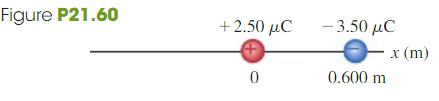# Problem: Two charges are placed on the x-axis: one of 2.5 μC at the origin and the other of -3.5μC at x =0.6 m. Find the position on the x-axis where the net force on a small charge +q would be zero

###### FREE Expert Solution

Coulomb's law:

$\overline{){\mathbf{F}}{\mathbf{=}}\frac{\mathbf{k}{\mathbf{q}}_{\mathbf{1}}{\mathbf{q}}_{\mathbf{2}}}{{\mathbf{r}}^{\mathbf{2}}}}$

The small charge +q is repelled by the charge at the origin and attracted by the charge at x = 0.6 m.

The charge cannot be placed between the two charges because both forces would act in the same direction.

89% (109 ratings)###### Problem Details

Two charges are placed on the x-axis: one of 2.5 μC at the origin and the other of -3.5μC at x =0.6 m. Find the position on the x-axis where the net force on a small charge +q would be zero